April 15th, 2021

## Today’s Class

1. Quick Review
• Out of Sample Performance $$\neq$$ In sample Performance
• AIC
• Stepwise
• LASSO
2. Cross Validation
3. Bias-Variance
4. Computational Notes
• Cross Validation
• Sparse Matrices
• LASSO with Factors
5. HW Discussion:
• HW2 Solutions
• HW3 setup

## Predictions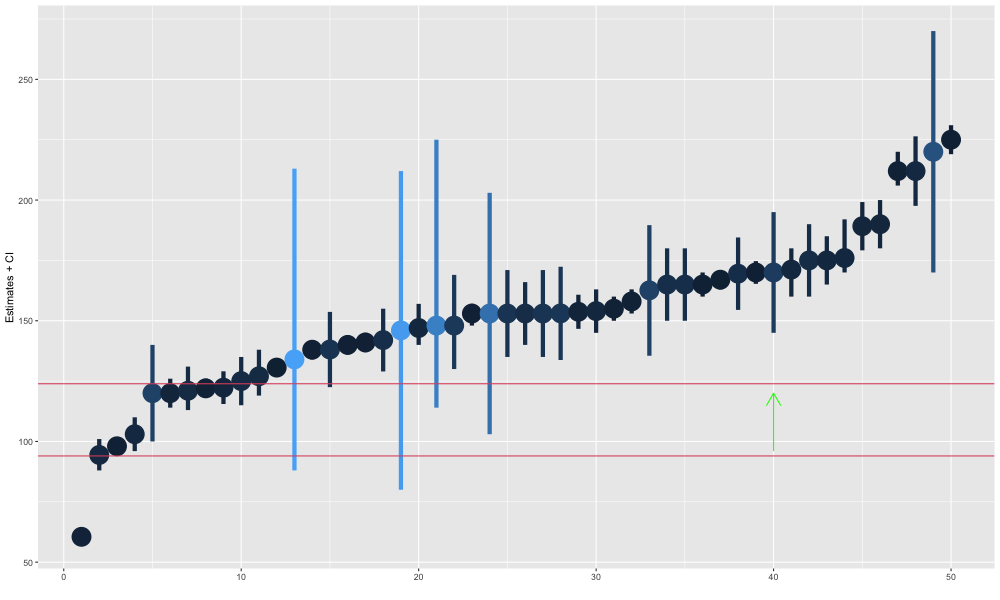## OOS performance

In sample, adding variables always improves predictive accuracy.

• IS $$R^2$$ for full model (200 vars): 56%
• IS $$R^2$$ for FDR cut model (25 vars): 18%

Out of Sample, we may gain predictive accuracy by dropping variables.

• OOS $$R^2$$ for the full model: -5.87.
• OOS $$R^2$$ for the cut model: 0.10.

THIS IS NEGATIVE. Out-of-Sample, we would be much BETTER off predicting the mean than with the full model.

OOS $$R^2$$ estimated with k-fold cross-validation.

## AIC

We can (with some assumptions) estimate OOS deviance using Akaike’s Information Criterion. For a model $$M$$ with $$k$$ variables estimated to be $$\hat{\beta}_M$$

$AIC = 2k + Dev(\hat{\beta}_M) = 2k - 2 log(L(\hat{\beta}_M|data))$ This was in our basic model output.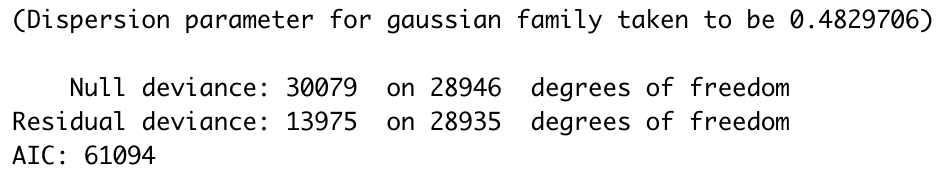This can help us compare models to choose one.

## Stepwise

But there are too many possible subsets of our variables to compare all of them. So we need some other method for coming up with a subset to compare.

Stepwise regression does this. It starts with a simple model, and “greedily” adds the best new variable repeatedly until adding a new variable no longer improves the AIC.

Because each choice depends on the current model parameters, small changes to the data can have big consequences for our model choices. This instability is bad for our OOS prediction.

## But AIC estimates OOS deviance/prediction errors

AIC estimates OOS deviance, and it does a good job of it, for a given model.

But once we started using AIC to choose our models, it ceased to be a good estimate of our deviance.

AIC was estimating the prediction errors of one model, not of a whole procedure which picks a model.

“When a measure becomes a target, it ceases to be a good measure” – Goodhart’s Law

We may encounter this problem again.

## LASSO

LASSO is the most commonly used regularized (or penalized) regression model. The lasso penalty is the $$l_1$$ norm of our parameters: $$pen(\beta) = \sum |\beta_j|$$, which penalizes larger coefficients and non-zero coefficients. So our estimates are: $\hat{\beta}_\lambda = \underset{\beta}{\text{argmin}}\left( Dev(\beta)+\lambda \sum_{j=1}^p |\beta_j|\right)$

## LASSO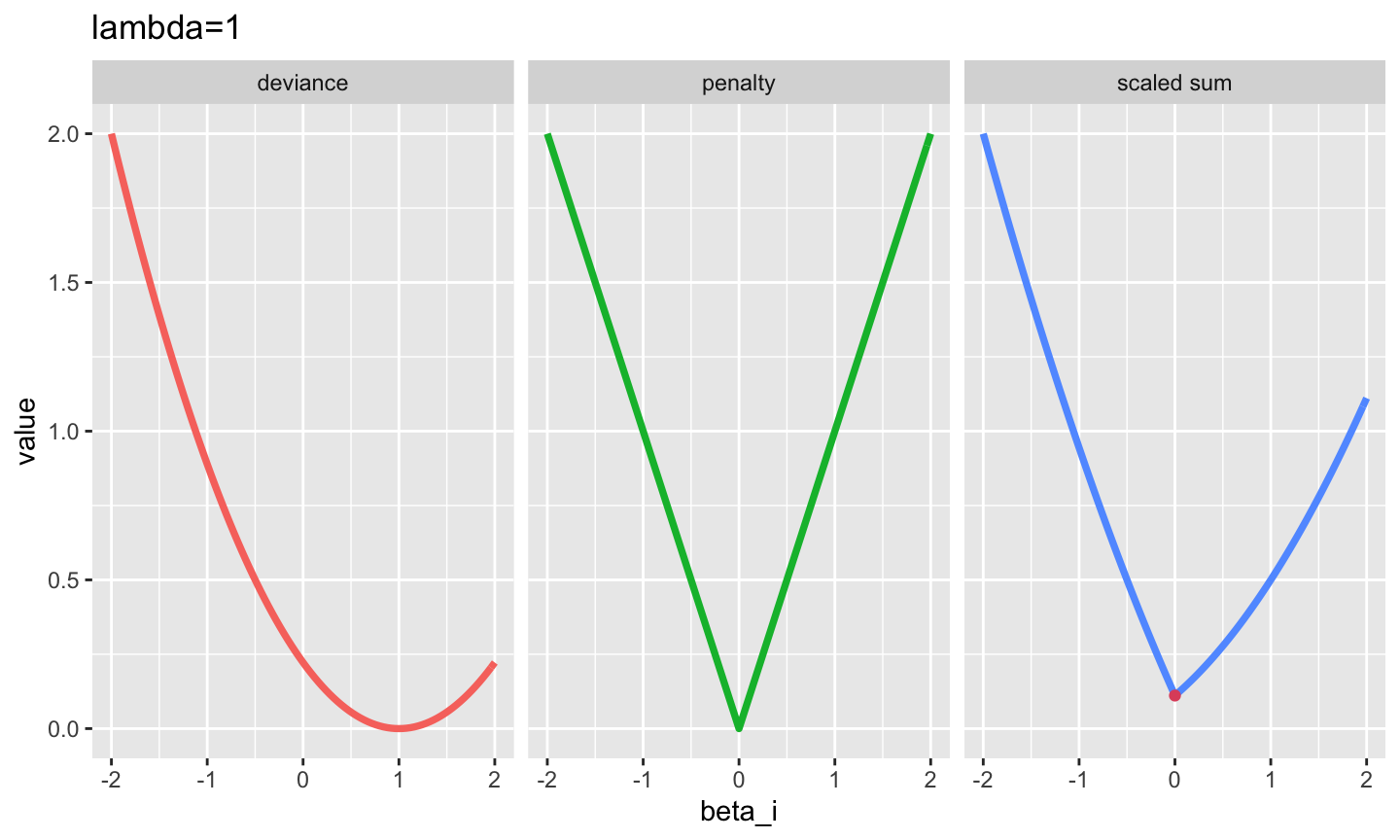## LASSO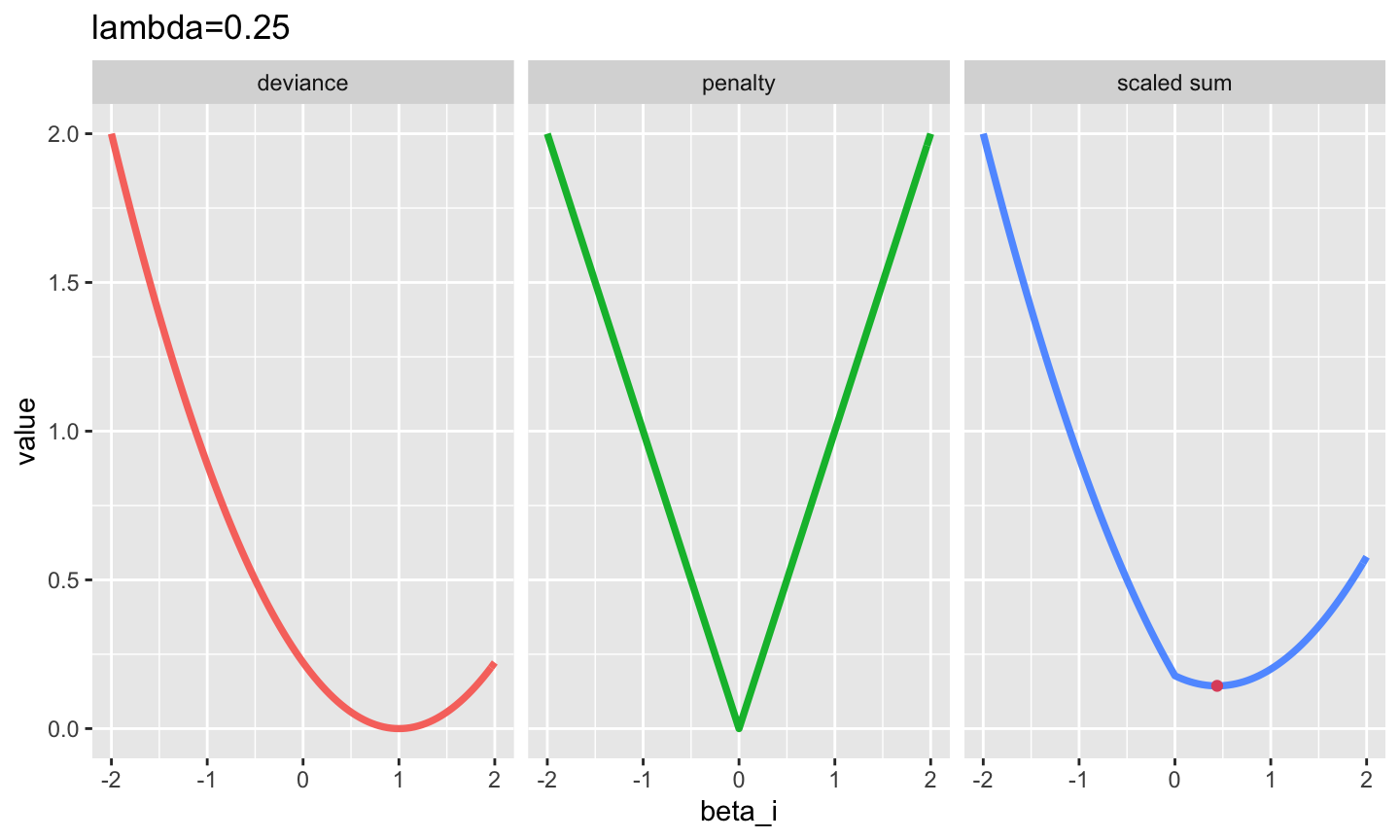## LASSO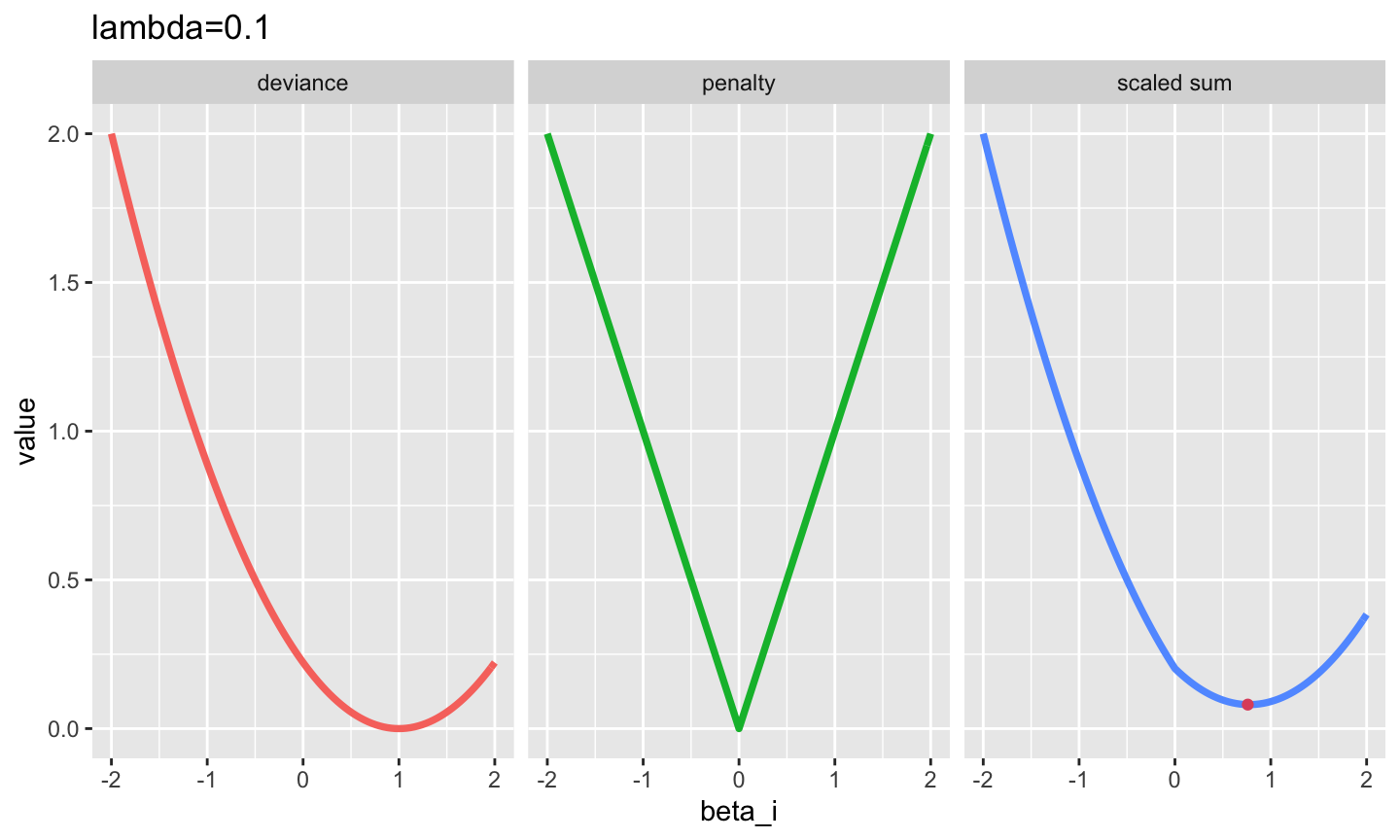## LASSO

We’ve framed LASSO as the solution to an optimization, given some weight parameter $$\lambda$$.

$\hat \beta _{LASSO} = \underset{\beta}{\text{argmin}} Dev(\beta)+\lambda \sum |\beta_j|$

It may be important, for reference to other places, to know that this can be rewritten as:

$\hat \beta_{LASSO} = \underset{\beta}{\text{argmin}} Dev(\beta) \quad s.t.\ \ \sum|\beta_j| = ||\beta||_1 \leq t$

Where the constraint $$t$$ is a bound on our $$l_1$$ norm. For any given dataset, there is a correspondence between $$\lambda$$ and $$t$$. So these representations are different ways of looking at the same problem.

## Recap

We could, like the bootstrap, repeat our testing-training procedure many times. But we want to guarantee each observation gets used as an ‘out-of-sample’ observation at least once.

Instead, we will “fold” the data. We will partition it (split into exclusive and exhaustive groups) into $$K$$ different groups of observations. Then, for k=1:K

• Use observations in group $$k$$ as test data.
• Train the models on the remaining data (for every $$\lambda$$)
• Predict the observations in group $$k$$ using those models
• Record the prediction errors for each lambda

This guarantees that each observation is left out once, and improves the performance of our routine.

## Picking $$K$$

There are several options:

• Leave-one-out Cross-validation: AKA $$K=n$$ is great, but much slower (fits every model under consideration $$n$$ times)
• $$K=5$$ corresponds to 5 different 20% leave-out samples.
• $$K=20$$ corresponds to 20 different 5% leave-out samples.

Most people set $$K \in [5,20]$$. I’ll mostly use 10.

$$\implies$$ Optimizing $$K$$ is very 3rd order. Not worth worrying about too much beyond time considerations and some preference for larger $$K$$.

## All Together.

We have a LASSO path indexed by $$\lambda_1 < \lambda_2 < ... < \lambda_T$$.

Cross-Validation for $$\lambda$$:
For each of $$k=1,...,K$$ folds:
1. Fit the path $$\hat\beta_{\lambda_1}^k,...,\hat\beta_{\lambda_T}^k$$ using the data not in fold $$k$$. 2. Get the fitted deviance for new data: $$-log\ P[y^k|X^k,\hat\beta_{\lambda_t}^k]$$ where $$k$$ denotes fold membership.

This gives us $$K$$ draws of the OOS deviance for each $$\lambda_t$$.

Choose the best $$\hat\lambda$$, and fit your model to all the data with that $$\hat\lambda$$.

## New Example: Comscore

This is from Comscore data. This data is about consumer spending on websites. We will try to predict household internet spending as a function of browser history.

Datanames:

• Covariates X: xweb
• outcomes Y: yspend

## In R

Again, Cross-validation is very easy and relatively fast for LASSO in R.

cv.spender = cv.glmnet(xweb,yspend)

And there is a nice plot for it too

## CV LASSO plot

plot(cv.spender)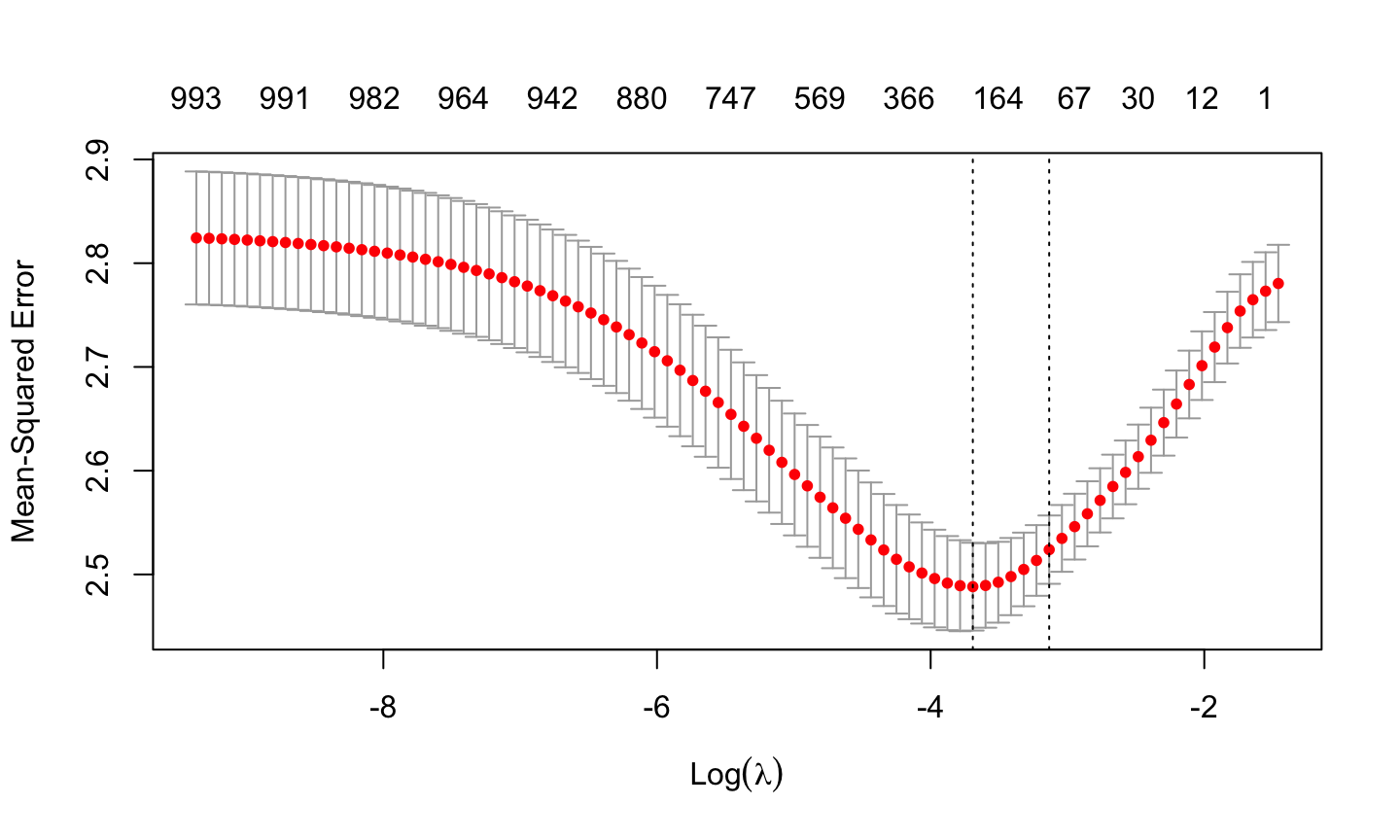## Mechanically What is Happening?

for (k in 1:K)

1. Estimate model on all data not in fold k
2. Calculate that model’s OOS prediction errors with data in fold k
3. Repeat for all models under consideration

So we have K estimates of deviance (MSE here) for each model. Now:

1. Estimate overall/mean deviance for each model.
2. Estimate our estimation error (standard errors) in that deviance.
3. Build CIs for Deviance

That plot shows the estimated mean/overall deviance for each lambda (across K) as a red point, and it shows the error bars on that estimate.

## CV LASSO plot

plot(cv.spender)